# How to Calculate and Solve for Total Oil Pore Volume of Reservoir Fluid Flow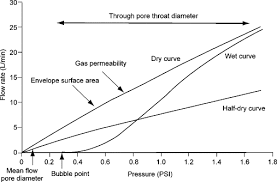The image above represents the total oil pore volume.

To compute for the total oil pore volume, four essential parameters are needed and these parameters are initial oil FVF (Boi), initial water saturation (Swi), oil in place (N) and gas cap ratio (m).

The formula for calculating the total oil pore volume:

(PV)o = NBoi(1 + m) / 1 + Swi

Where;

(PV)o = Total Oil Pore Volume
Boi = Initial Oil FVF
Swi = Initial Water Saturation
N = Oil in Place
m = Gas Cap Ratio

Let’s solve an example;
Find the total oil pore volume when the initial oil FVF is 9, the initial water saturation is 11, the oil in place is 21 and the gas cap ratio is 18.

This implies that;

Boi = Initial Oil FVF = 9
Swi = Initial Water Saturation = 11
N = Oil in Place = 21
m = Gas Cap Ratio = 18

(PV)o = NBoi(1 + m) / 1 + Swi
(PV)o = 21 x 9(1 + 18) / 1 + 11
(PV)o = 3591 / -10
(PV)o = -359.1

Therefore, the total oil pore volume is -359.1

Nickzom Calculator – The Calculator Encyclopedia is capable of calculating the total oil pore volume.

To get the answer and workings of the total oil pore volume using the Nickzom Calculator – The Calculator Encyclopedia. First, you need to obtain the app.

You can get this app via any of these means:

To get access to the professional version via web, you need to register and subscribe for NGN 1,500 per annum to have utter access to all functionalities.
You can also try the demo version via https://www.nickzom.org/calculator

Apple (Paid) – https://itunes.apple.com/us/app/nickzom-calculator/id1331162702?mt=8
Once, you have obtained the calculator encyclopedia app, proceed to the Calculator Map, then click on Petroleum under EngineeringNow, Click on Reservoir Fluid Flow under Petroleum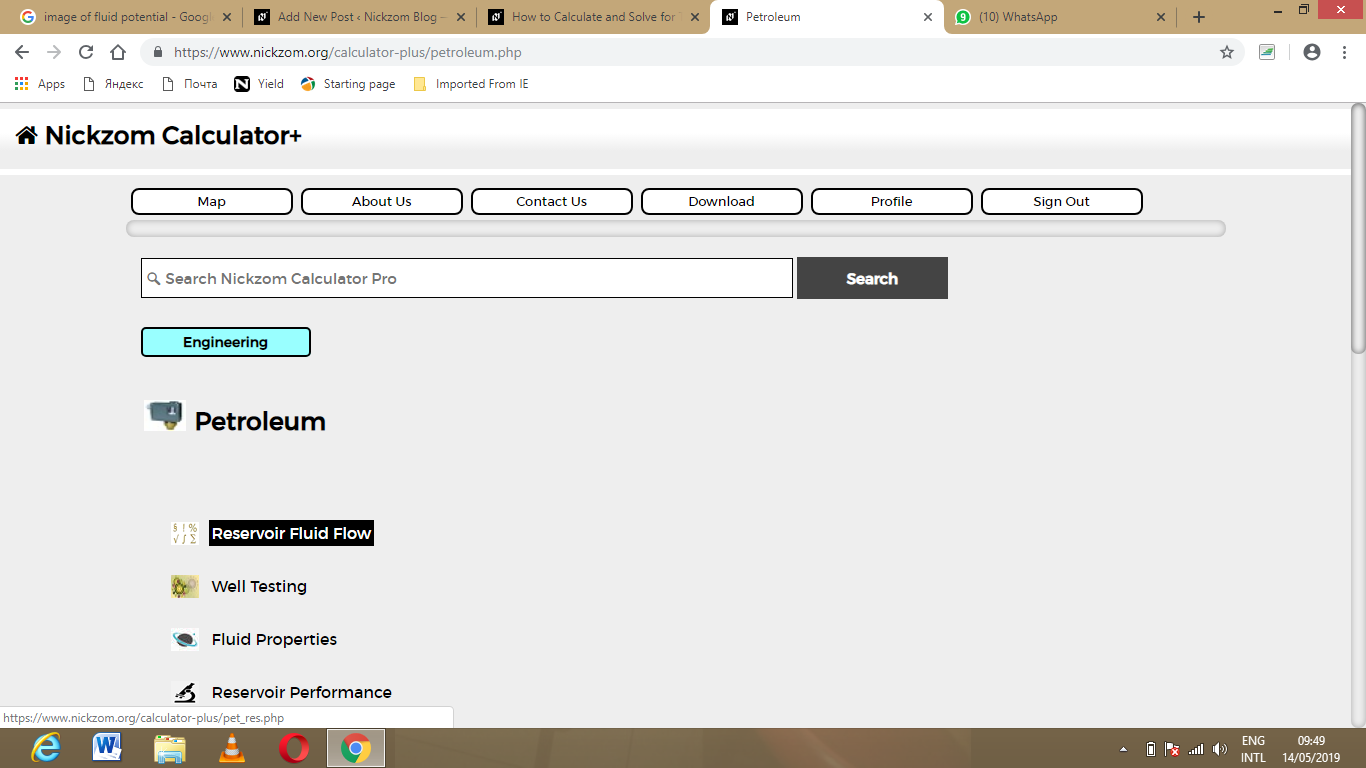Now, Click on total oil pore volume under Reservoir Fluid Flow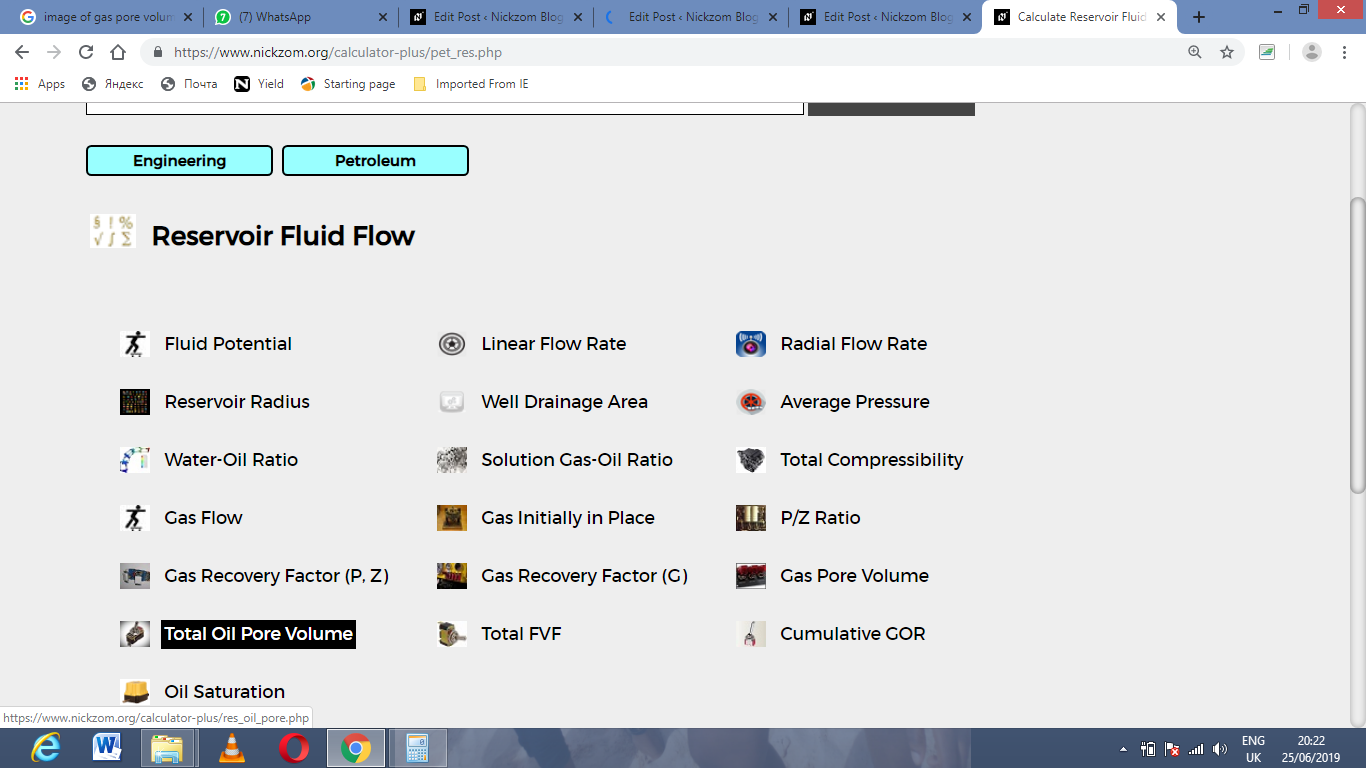The screenshot below displays the page or activity to enter your value, to get the answer for the total oil pore volume according to the respective parameter which are the initial oil FVF (Boi), initial water saturation (Swi), oil in place (N) and gas cap ratio (m).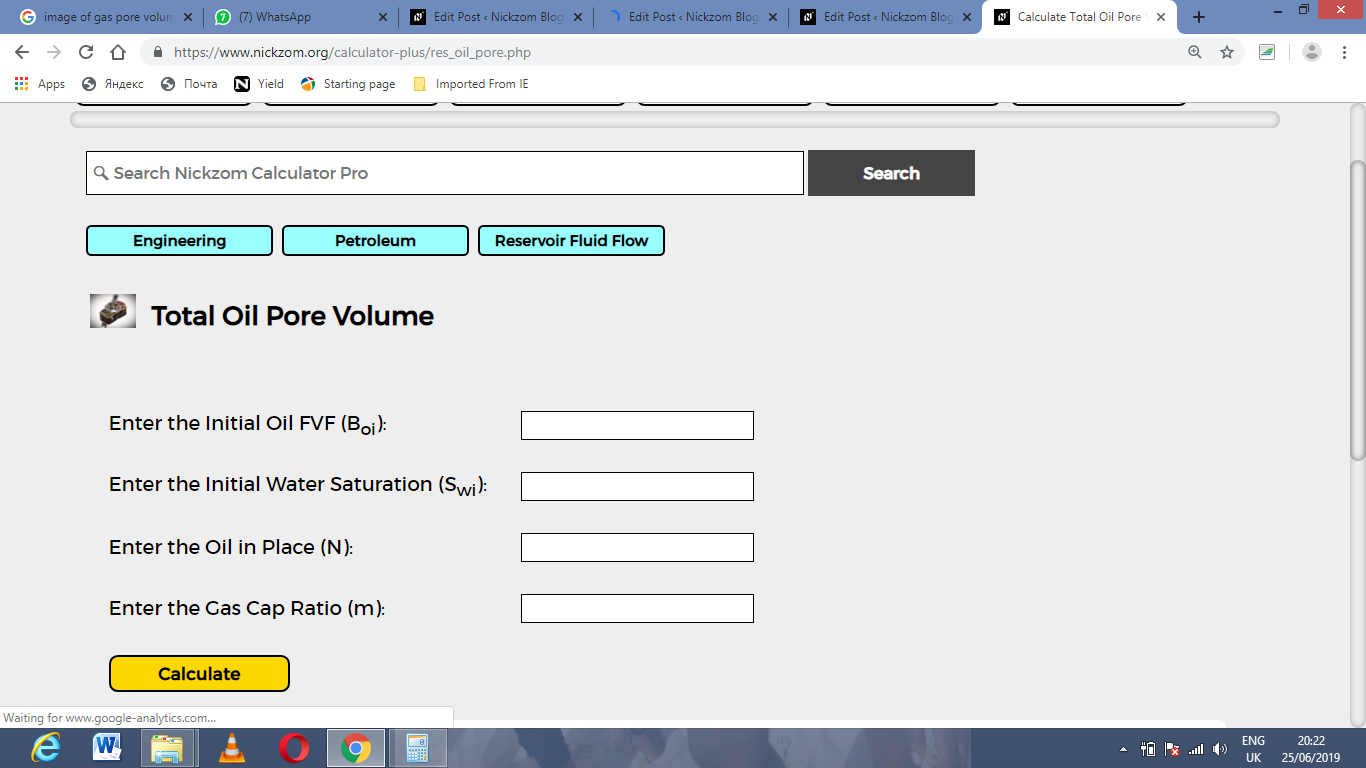Now, enter the value appropriately and accordingly for the parameter as required by the initial oil FVF (Boi) is 9, initial water saturation (Swi) is 11, oil in place (N) is 21 and gas cap ratio (m) is 18.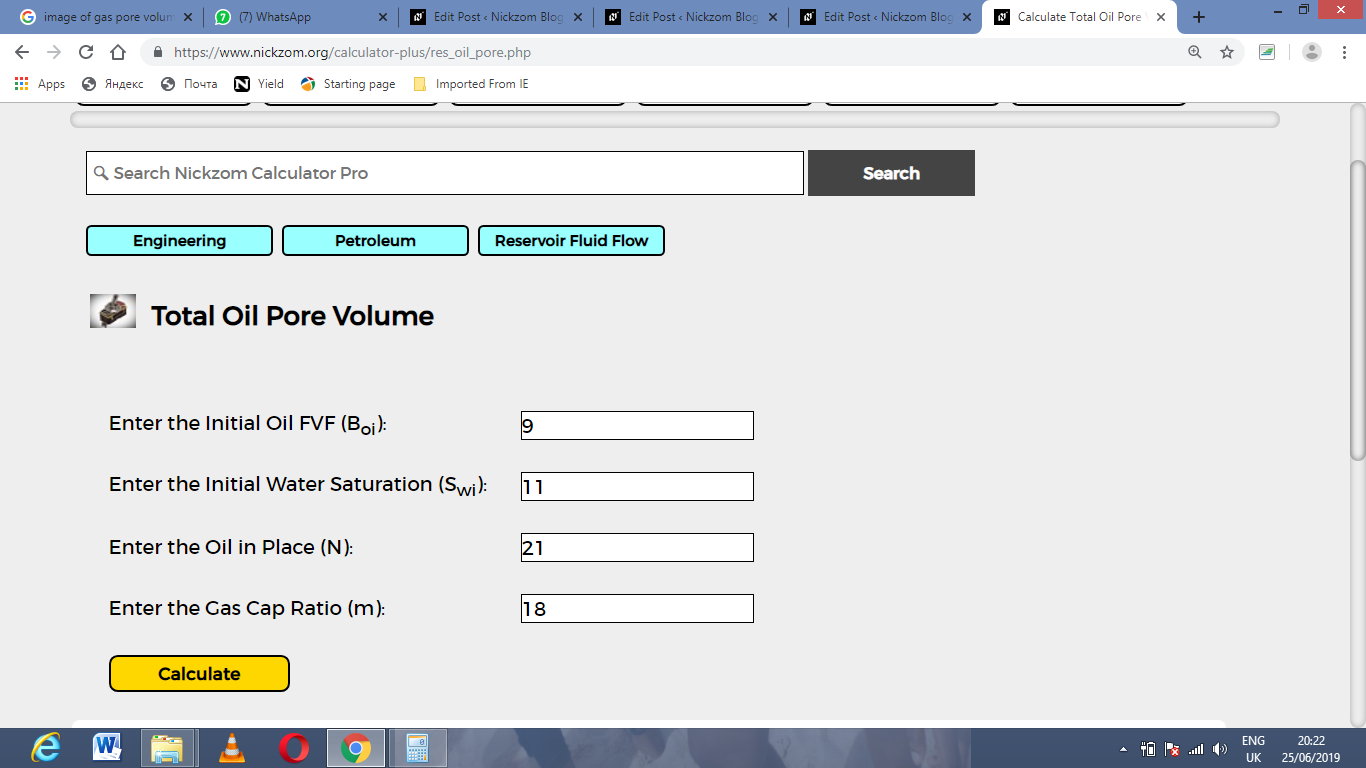Finally, Click on Calculate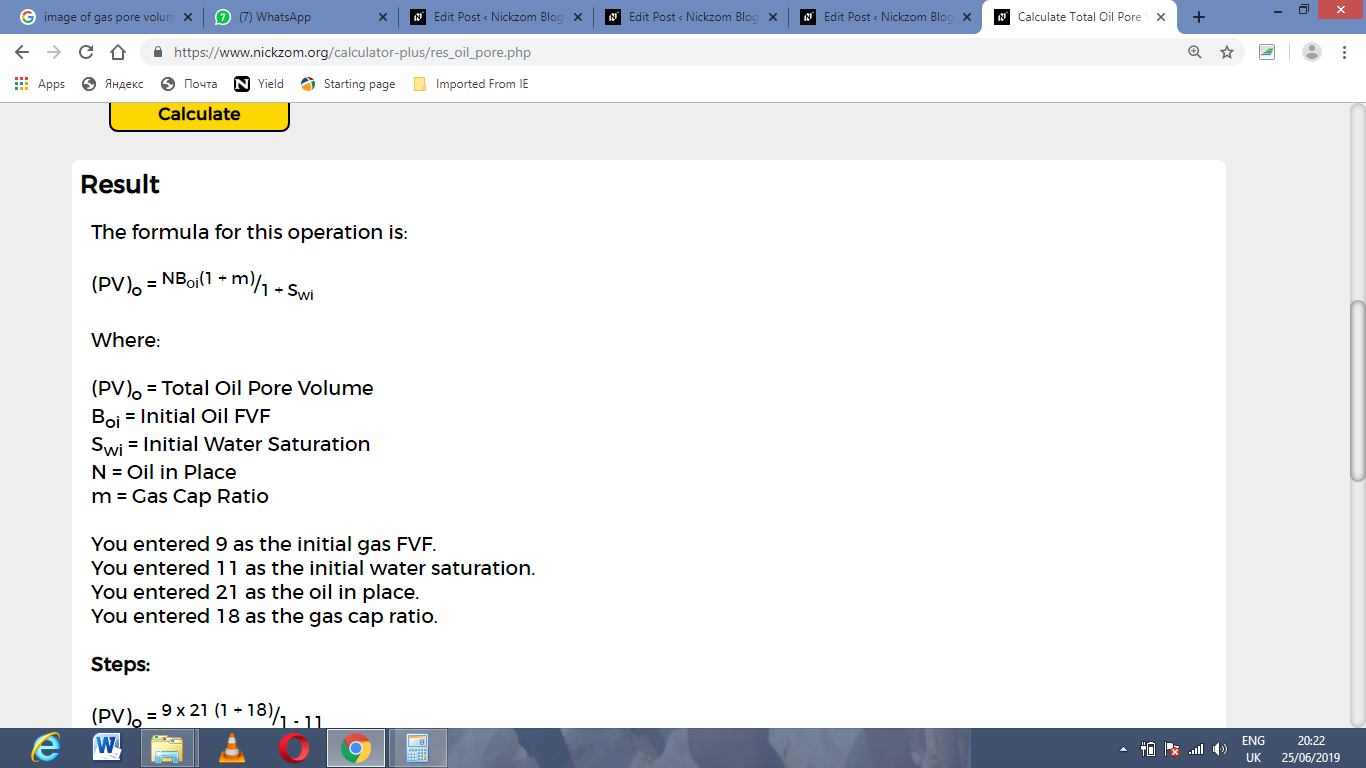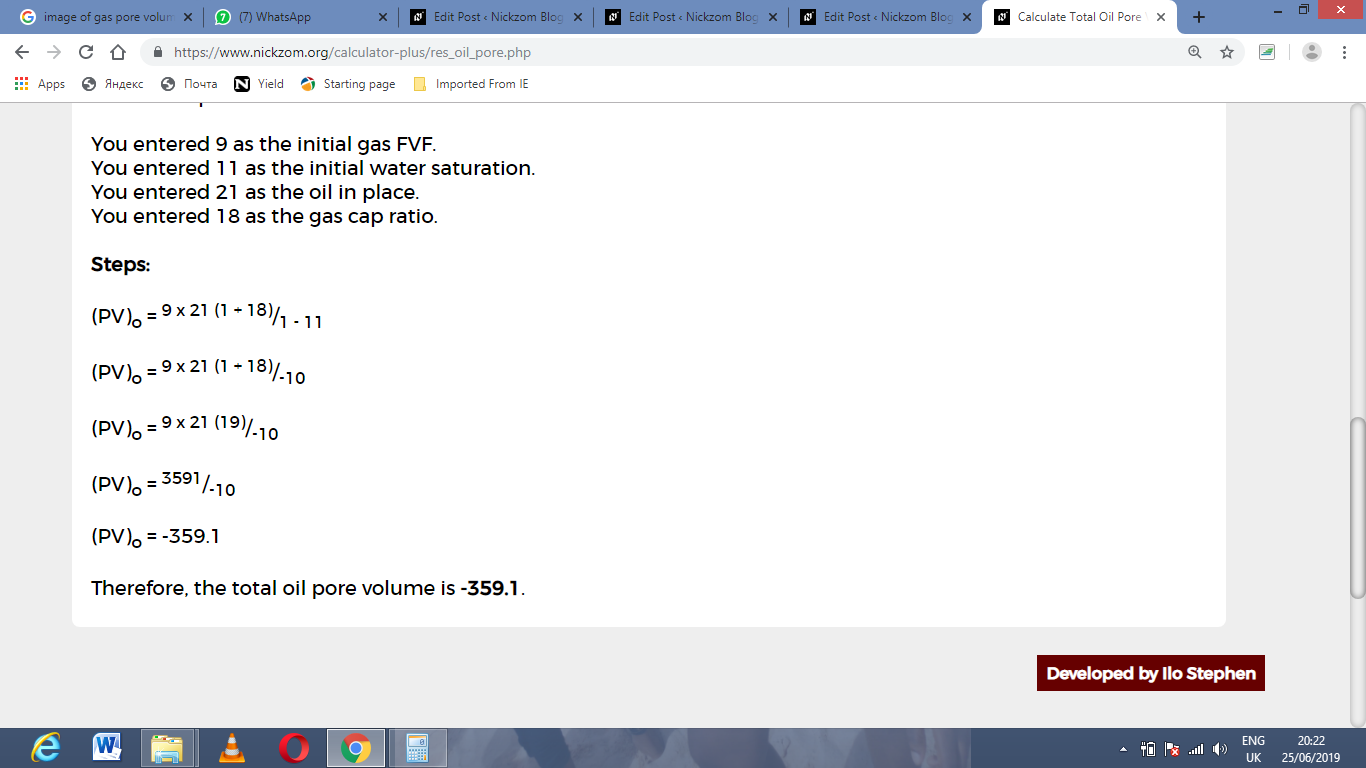As you can see from the screenshot above, Nickzom Calculator– The Calculator Encyclopedia solves for the total oil pore volume and presents the formula, workings and steps too.